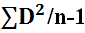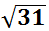# Sample Variance and Standard Deviation

## Introduction

• Ram, Arjun, Ravi, Ashok are three friends of the same class and they are awaiting their exam results. All of them got 85% marks which were shocking as their response to the different papers was different, few had written English very well and few had written it bad. So how did this happen?
• Let us have a look at their scorecard of individual subjects which is out of 100:
Subject/Student English Hindi Maths Science Total percentage
Ram 70 96 85 89 85
Arjun 99 45 99 97 85
Ravi 82 88 80 90 85
Ashok 60 82 99 99 85
• It is well known that the total percentage is the average percentage of each subject. Though the total percentage of all the four is the same, the scoring pattern of each of them is very different from each other.
• Hence, Average or Mean gives us detail about the overall picture only and it skips the individual contribution of all the elements.
• In other words, Average gives us information about the size or value of elements of the dataset (total percentage) and not about the spread of the values in elements i.e. how much or how less is the contribution of an element (percentage in each subject).
• The measure of dispersion helps us to overcome the drawback of the Mean observed above, it helps in understanding the contribution of each element in a dataset.
• Dispersion is a measure to find out the extent to which values on element differ from the Mean of dataset i.e. in the above example measure of dispersion will give us an idea that how much score did Ram got in each subject (how much or how less than 85).
• The population is the collection of specified groups of similar objects based on some common parameters. E.g. Residents of State of Maharashtra or All tigers in a Tiger Reserve
• The members of the population are known as Elements of the Population. e.g. Tiger is the element of a population that is defined as all tigers of the Tiger Reserve. The total number of elements in a Population is known as Population Size.
• It is very difficult and time-consuming to apply analysis on a population, hence few elements are taken from the population to form a sample for analysis purposes, in other words a Sample is a subset of the population. E.g. Few Tigers were selected from the tiger reserve for the purpose of health check-up, here the few tigers examined to form a sample. A total number of elements in a Sample is known as Sample Size.
• There are various ways to measure the dispersion of a dataset which we will study in upcoming sections.

## Sample Standard Deviation

• Standard Deviation is the most widely used method to measure the Dispersion of a set of data. It measures the deviation of elements of a sample/dataset from its mean.
• The formula for calculation of Standard Deviation of Sample is:
• Sample Standard Deviation• Where D is the difference between the elements of a sample and its mean
• And n is the sample size.

#### Example 1:

• Let us take a sample: 2,5,8,10,12,15,18 and Calculate its Standard Deviation
• Solution:
• Step 1: Calculating Mean:
• Mean = (2+5+8+10+12+15+18)/7 = 70/7 = 10
• Step 2: Calculating the Deviation of Mean and it's Square:
Element Deviation from Mean ‘D’ D2
2 -8 64
5 -5 25
8 -2 4
10 0 0
12 2 4
15 5 25
18 8 64
• Step 3: Calculating• Step 4: Taking Square Root of the Mean of Square of Deviations:
• Sample Standard Deviation == 5.567
• Standard Deviation depends on each value of the sample, change in any of the values affects the Standard Deviation.
• Standard Deviation is easy to interpret, the rule of standard deviation says that almost all elements of a sample/dataset should lie within Mean ± 3* Standard Deviation.

## Sample Variance